The Research Seminar in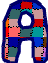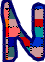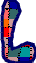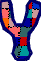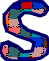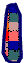meets in S230 on Tuesday, February 26, 2002, 1 pm. All interested faculty and students are invited to attend.Martin Bohner will talk on   Formulas of Bendixson and Alekseev for Difference Equations. Abstract: A well-known formula of Bendixson states that solutions of first order differential equations, as functions of their initial conditions, satisfy a certain partial differential equation. A consequence is Alekseev's nonlinear variation of parameters formula. In this talk we prove corresponding results for difference equations. To achieve this, we make use of the recently introduced concept of alpha derivatives rather than of differences or usual derivatives. This technique allows us to also offer generalizations of our results to alpha dynamic equations, which include among others ordinary differential and difference equations.